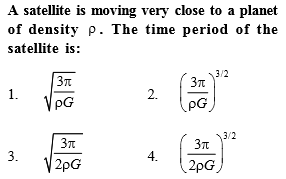# NEET Questions SolvedConcept Videos :-

#1 | Kepler's 1st Law
#2 | Kepler's 2nd Law
#3 | Kepler's 3rd Law

Concept Questions :-

Kepler laws

$T=\frac{2\mathrm{\pi R}}{v}=\frac{2\mathrm{\pi R}}{\sqrt{\frac{GM}{R}}}=\frac{2{\mathrm{\pi R}}^{3}{2}}}{\sqrt{GM}}\phantom{\rule{0ex}{0ex}}T=\frac{2\pi {R}^{3}{2}}}{\sqrt{G\frac{4}{3}{\mathrm{\pi R}}^{3}\mathrm{\rho }}}=\frac{2{\mathrm{\pi R}}^{3}{2}}}{2\sqrt{\frac{\mathrm{\pi }}{3}\rho G}{R}^{3}{2}}}=\sqrt{\frac{3\mathrm{\pi }}{\rho G}}$

Difficulty Level:

• 46%
• 26%
• 20%
• 10%
Crack NEET with Online Course - Free Trial (Offer Valid Till September 17, 2019)